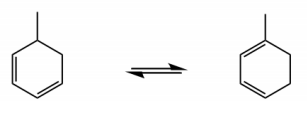Ch. 16 - Conjugated SystemsWorksheetSee all chapters
 Ch. 1 - A Review of General Chemistry 4hrs & 47mins 0% complete Worksheet Ch. 2 - Molecular Representations 1hr & 12mins 0% complete Worksheet Ch. 3 - Acids and Bases 2hrs & 45mins 0% complete Worksheet Ch. 4 - Alkanes and Cycloalkanes 4hrs & 18mins 0% complete Worksheet Ch. 5 - Chirality 3hrs & 33mins 0% complete Worksheet Ch. 6 - Thermodynamics and Kinetics 1hr & 19mins 0% complete Worksheet Ch. 7 - Substitution Reactions 1hr & 46mins 0% complete Worksheet Ch. 8 - Elimination Reactions 2hrs & 24mins 0% complete Worksheet Ch. 9 - Alkenes and Alkynes 2hrs & 10mins 0% complete Worksheet Ch. 10 - Addition Reactions 3hrs & 33mins 0% complete Worksheet Ch. 11 - Radical Reactions 1hr & 57mins 0% complete Worksheet Ch. 12 - Alcohols, Ethers, Epoxides and Thiols 2hrs & 34mins 0% complete Worksheet Ch. 13 - Alcohols and Carbonyl Compounds 2hrs & 14mins 0% complete Worksheet Ch. 14 - Synthetic Techniques 1hr & 28mins 0% complete Worksheet Ch. 15 - Analytical Techniques: IR, NMR, Mass Spect 7hrs & 18mins 0% complete Worksheet Ch. 16 - Conjugated Systems 5hrs & 49mins 0% complete Worksheet Ch. 17 - Aromaticity 2hrs & 24mins 0% complete Worksheet Ch. 18 - Reactions of Aromatics: EAS and Beyond 4hrs & 31mins 0% complete Worksheet Ch. 19 - Aldehydes and Ketones: Nucleophilic Addition 4hrs & 54mins 0% complete Worksheet Ch. 20 - Carboxylic Acid Derivatives: NAS 2hrs & 3mins 0% complete Worksheet Ch. 21 - Enolate Chemistry: Reactions at the Alpha-Carbon 1hr & 59mins 0% complete Worksheet Ch. 22 - Condensation Chemistry 2hrs & 13mins 0% complete Worksheet Ch. 23 - Amines 1hr & 43mins 0% complete Worksheet Ch. 24 - Carbohydrates 5hrs & 56mins 0% complete Worksheet Ch. 25 - Phenols 15mins 0% complete Worksheet Ch. 26 - Amino Acids, Peptides, and Proteins 2hrs & 54mins 0% complete Worksheet

Here we will discuss an intramolecular pericyclic reaction in which 0 pi bonds are destroyed after a cyclic mechanism.

Concept #1: Definition of Sigmatropic Shifts

Transcript

In this video we're going to discuss a type of pericyclic reaction called a Sigmatropic shift. So, what is a Sigmatropic shift? Well, it's an intramolecular pericyclic reaction in which 0 pi bonds are destroyed after a cyclic mechanism. So, we know this by definition. Remember, that Sigmatropic shifts were the type of pericyclic reactions that occur with no pi bonds being changed between the reactant and the product and that's exactly what we're going to see in this example I will get to this example in just a second but let me give you a few more tips about what a sigmatropic shift is so the pi bonds aren't going to change but what it does involve every single time is the breaking of one sigma bond. So, a sigma bond is going to break and then the making of a sigma bond. So, what we're basically doing is that the double bonds aren't changing, in fact, they do change position but they're not changing in terms of quantity, the Sigma bonds aren't changing in quantity either but it's important to note that that's actually a very big change that happens here, which is that one Sigma bond is destroyed and another Sigma bond is created. So, what that means is that these reactions are usually going to take the form of numerous types of rearrangements, usually you're not going to see a very distinct difference between the product and the reactant where they look very different from each other it's usually just that something's going to rearrange or shift and you're going to say huh I wonder what happened and afterwards by looking at counting the number of pi bonds you can tell this must have been a sigmatropic shift, okay? Because of that the products are typically constitutional isomers of the reactant. So, what that means is again nothing's changing, no atoms are changing but the way that they're connected to each other is changing because you're making bonds in you're breaking bonds. So, usually there's going to be constitutional isomers of each other, very common examples of these, which you may or may not have to know based on your class and your professor but some very, very common example are the Cope Rearrangement and the claisen rearrangement, we'll go on to learn these in depth if it's something that your professor and your textbook requires but I'm just letting you know that even if you don't need to know them these are examples of sigmatropic shifts okay, cool. So, let's look at this reaction and try to figure out the mechanism. So, once again, we have this is a heat-activated reaction where I'm basically starting off with 2 pi bonds and I'm ending up with again 2 pi bonds. So, we notice the Sigma tropic shift because this is a constitutional isomer of the first one but no pi bonds changed, okay? Now, let's try to figure out what would be a mechanism that would make sense here, how could we create something like this, right? And guys it's once again is going to be cyclic. So, we're going to see is that you're breaking the bond and you're making a bond. So, in this case, which is the bond that we're breaking, we're breaking the one that was right here, okay? So, it's actually where we want to start our arrows from not that you have to because it's concerted. So, you can start from anywhere but just to think about it logically it makes sense that you would take these electrons and make a double bond here, then you would take this pi bond and make a new sigma bond here and then if you make that bond you need to break this bond and put the double one there. So, once again, we have this pericyclic concerted reaction but it's leading to just the formation of a constitutional isomer, okay? So, in the next video we're going to talk about how do you name and identify sigmatropic shifts.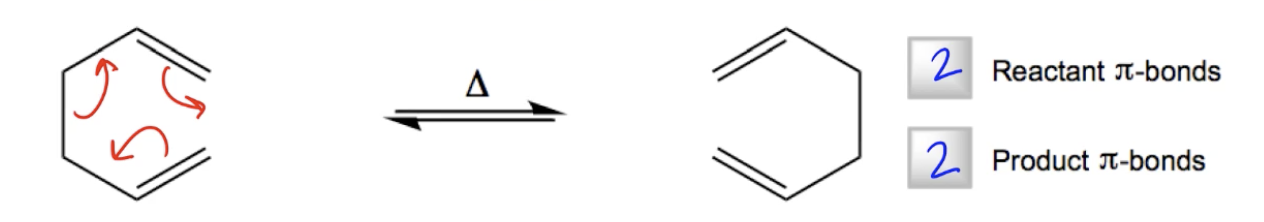Concept #2: Nomenclature of Sigmatropic Shifts

Transcript

Alright, so there are numerous types of sigmatropic shifts out there, some of them have common names like the cope Shift, the cope Rearrangement or the claisen rearrangement but the most important thing about naming sigmatropic shift isn't memorizing specific names, it's how to identify the actual nomenclature of the reaction. So, that's what I'm going to teach you right now, thankfully there's a shared nomenclature amongst all Sigmatropic shifts and that's what we're going to learn. Now, and I'm going to teach you how to apply this nomenclature to the example that we just saw above, so the Sigmatropic shift is always referred to as an x, y Sigmatropic shift, where x and y are different numbers of atoms, okay? So, what does this mean? Well, the first sigma bond that's broken. So, remember that you're always breaking a bond and you're making a bond, right? So, the first one that's broken is going to be considered atom one. So, the atom that the bond is being broken on is going to receive the title of one. Now, this is not, this has nothing to do with iupac nomenclature, so this is not us trying to name the compound from scratch, this is just trying to name the reaction that's happening, atom one would be where the position where a break has happened or the destruction of a bond has happened. So, then what's the x, y that has to do with where the new Sigma bond is created, the position that the new Sigma bond is created would be the x and the y, okay? So, basically what you would do is you would say that you are taking the electrons from one atom and you're putting them in between these other two atoms and those other two atoms are going to be your x and y, okay?

So, let's go ahead and look at an example up here, where we have once again our Sigmatropic shift. So, which one is the bond that was being broken? what do you see? what's the bond that was being broken? it was this one, so that means that both of these atoms get labeled as one, okay? Because in this case I broke in the middle of a chain I broke it upon, right in the middle of the chain. So, both of those receive the number 1, okay? Now, does that mean that my numbers are 1, 1? no, because for the x and y part we have to look at where was a bond made. So, where was the bond made? Well, let's see, a new bond appears to have made to have been made here. So, what we have to do is we have to count from both chains, what atoms, what's the number of atom that it would take to get to that position. So, for the top one it would be 2 and then 3, for the bottom it would be 2 and then 3, so, the bond that was broken was 1 and the new bond was created in between atom 3 and atom 3. So, what that means is that this is going to be a 3, 3 sigmatropic shift, cool? Awesome. So, now we just did one example and now what we're going to do is we're going to do four more examples of common types of shifts, try to do it first and then I'm going to tell you the right answer.

Naming Summary:

• Always described as [x,y]-sigmatropic shifts
1. σ–bond broken = Atom 1
2. σ–bond created = Atoms [x,y]

Practice: Provide the correct names and mechanisms for the following sigmatropic shifts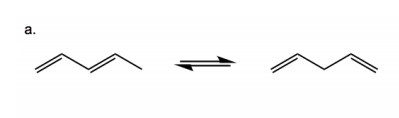Practice: Provide the correct names and mechanisms for the following sigmatropic shifts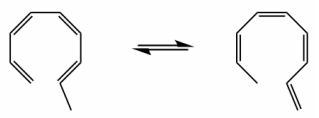Practice: Provide the correct names and mechanisms for the following sigmatropic shifts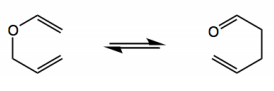Practice: Provide the correct names and mechanisms for the following sigmatropic shifts